• 复杂度分析第一次写博文，侵删并见谅为什么需要复杂度分析大O复杂度表示法时间复杂度分析几种常见时间复杂度实例分析空间复杂度分析 第一次写博文，侵删并见谅 以下开始正文 为什么需要复杂度分析 把代码跑一遍，...


复杂度分析
第一次写博文，侵删并见谅为什么需要复杂度分析大O复杂度表示法时间复杂度分析几种常见时间复杂度实例分析空间复杂度分析

第一次写博文，侵删并见谅
以下开始正文
为什么需要复杂度分析
把代码跑一遍，通过统计、监控，就能得到算法执行的时间和占用的内存大小，而且是非常精准的，那为什么还要做时间、空间复杂度分析呢？ 原因有二：
测试结果非常依赖测试环境： 例如同样的代码在 i9 和 i3 上跑的时间会不一样测试结果受数据规模的影响很大： 小规模数据 情况下 插入排序O(n²) 可能比 快速排序O(nlogn) 还要快 结果: 我们需要一个不用具体的测试数据来测试,就可以粗略地估计 算法的执行效率的方法. 这就是我们今天要讲的时间、空间复杂度分析方法。
大O复杂度表示法
例1.
1. int cal(int n) {
2. 	int sum = 0;
3. 	int i = 1;
4. 	for (; i <= n; ++i) {
5. 		sum = sum + i;
6.	 }
7. 	return sum;
8. }

我们假设每行代码执行的时间为unit-time 第2、3行代码分别需要1个unit_time的执行时间， 第4、5行都运行了n遍， 所以需要2n*unit_time， 所以这段代码执行的时间就是T(n) = (2n+2)*unit_time。
例2.
 1. int cal(int n) {
2. 	int sum = 0;
3. 	int i = 1;
4. 	int j = 1;
5. 	for (; i <= n; ++i) {
6. 		j = 1;
7. 		for (; j <= n; ++j) {
8. 			sum = sum + i * j;
}
}
}

我们依然假设每行代码执行的时间为unit-time 第2、 3、 4行代码，每行都需要1个unit_time的执行时间 第5、 6行代码循环执行了n遍，需要2n * unit_time的执行时间 第7、 8行代码循环执行了n2遍，所以需要2n2 * unit_time的执行时间 所以，整段代码总的执行时间T(n) = (2n2+2n+3)*unit_time 注意，大O就要登场了

T

(

n

)

=

O

(

f

(

n

)

)

T(n) = O(f(n))

T(n)表示代码执行的时间n表示数据规模f(n)表示每行代码执行的次数总和
大O时间复杂度实际上并不具体表示代码真正的执行时间， 而是代码执行时间随数据规模增长的变化趋势， 所以也叫作渐进时间复杂度， 简称时间复杂度。
低阶、常量、系数三部分并不左右增长趋势，所以例1的T(n) = O(n), 例2的T(n) = O(n2)
时间复杂度分析
三个实用方法：
只关注循环执行次数最多的一段代码 例1和例2  加法法则  乘法法则
几种常见时间复杂度实例分析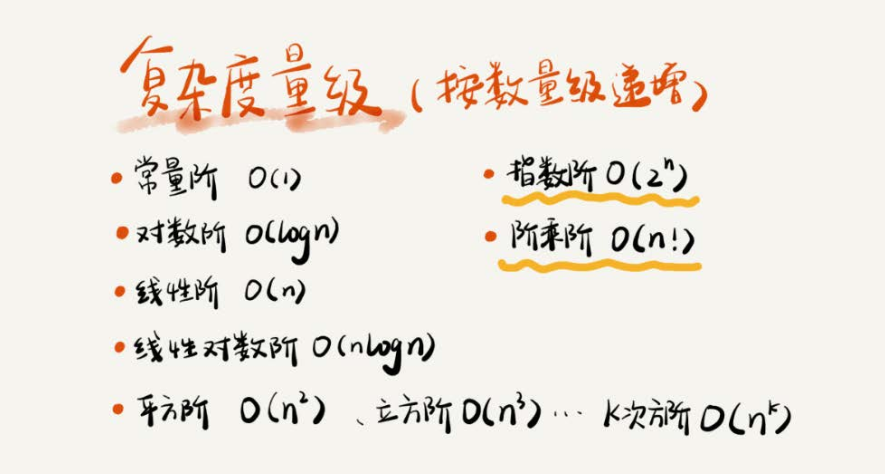空间复杂度分析
展开全文数据结构 算法
• ## 递归算法时间复杂度分析

万次阅读 多人点赞 2018-09-17 16:16:59
递归算法时间复杂度分析 时间复杂度： 一般情况下，算法中基本操作重复的次数就是问题规模n的某个函数f（n），进而分析f（n）随n的变化情况并确定T（n）的数量级。这里用‘o’来表示数量级，给出算法时间复杂度。 T...
                             递归算法时间复杂度分析
时间复杂度：
一般情况下，算法中基本操作重复的次数就是问题规模n的某个函数f（n），进而分析f（n）随n的变化情况并确定T（n）的数量级。这里用‘o’来表示数量级，给出算法时间复杂度。  T（n）=o（f（n））；  它表示随问题规模n的增大，算法的执行时间增长率和f（n）增长率成正比，这称作算法的渐进时间复杂度。而我们一般情况下讨论的最坏的时间复杂度。  空间复杂度：  算法的空间复杂度并不是实际占用的空间，而是计算整个算法空间辅助空间单元的个数，与问题的规模没有关系。算法的空间复杂度S（n）定义为该算法所耗费空间的数量级。  S（n）=o（f（n））  若算法执行所需要的辅助空间相对于输入数据n而言是一个常数，则称这个算法空间复杂度辅助空间为o（1）；  递归算法空间复杂度：递归深度n*每次递归所要的辅助空间，如果每次递归所需要的辅助空间为常数，则递归空间复杂度o（n）。
递归算法分析
1、利用数列知识
累加法：递推关系式为an+1−an=f(n)an+1−an=f(n)采用累加法。累乘法：递推关系式为an+1an=f(n)an+1an=f(n)采用累乘法。构造法：递推关系式为(1)aa+1=pan+qaa+1=pan+q，(2)aa+1=pan+qnaa+1=pan+qn，都可以通过恒等变形，构造出等差或等比数列，利用等差或等比数列的定义进行解题，其中的构造方法可通过待定系数法来进行。和化项法：递推公式为Sn=f(n)Sn=f(n)或Sn=f(an)Sn=f(an)一般利用 an={S1，Sn−Sn−1，当n=1当n>=2an={S1，当n=1Sn−Sn−1，当n>=2 用特征方程求解递推方程（感觉比较生僻，不做解释）迭代法： 从原始递推方程开始，反复将对于递推方程左边的函数用右边的等式代入，直到得到初值，然后将所得的结果进行化简。  例如在调用归并排序mergeSort(a,0,n-1)对数组a[0...n−1]a[0...n−1]排序时，执行时间T(n)T(n)的递推关系式为： T(n)={O(1)，2T(n2)+O(n)，当n=1当n>=2T(n)={O(1)，当n=12T(n2)+O(n)，当n>=2  其中，O(n)O(n)为merge()所需要的时间，设为cncn（c为正常量）。因此：  T(n)=2T(n2)+cn=2(2T(n4)+cn2)+cn=22T(n4)+2cn=23T(n8)+3cn=...=2kT(n2k)+kcn=nO(1)+cnlog2n=O(nlog2n),(假设n=2k,则k=log2n)T(n)=2T(n2)+cn=2(2T(n4)+cn2)+cn=22T(n4)+2cn=23T(n8)+3cn=...=2kT(n2k)+kcn=nO(1)+cnlog2⁡n=O(nlog2⁡n),(假设n=2k,则k=log2⁡n)

忽略求解细节。在我们求解递归式时，因为最终是要求得一个时间上限，所以在求解时常常省略一些细节。比如mergeSort(a,0,n-1)运行时间的实际递归式应该是：
T(n)={O(1)，T(⌈n2⌉)+T(⌊n2⌋)+O(n)，当n=1当n>=2T(n)={O(1)，当n=1T(⌈n2⌉)+T(⌊n2⌋)+O(n)，当n>=2
但我们忽略这些上取整、下取整以及边界条件，甚至假设问题规模n=2kn=2k，这都是为方便求解而忽略的细节。经验和一些定理告诉我们，这些细节不会影响算法时间复杂度的渐近界。

类似的，我们也可以用迭代法求解汉诺塔递归求解时的时间复杂度。但遗憾的是，迭代法一般适用于一阶的递推方程。对于二阶及以上（即T(n)依赖它前面更多个递归项T(n)依赖它前面更多个递归项）的递推方程，迭代法将导致迭代后的项太多，从而使得求和公式过于复杂，因此需要将递推方程化简，利用差消法等技巧将高阶递推方程化为一阶递推方程。如在求快速排序算法平均时间复杂度T(n)T(n)的递推方程，T(n)T(n)依赖T(n−1)、T(n−2)、...、T(1)T(n−1)、T(n−2)、...、T(1)等所有的项，这样的递推方程也称为全部历史递推方程。（这里省略快速排序算法平均复杂度T(n)的求解过程）
小结：上面6种递推关系是高中、本科知识，在此重点介绍了迭代法，其它几种方法虽未在本篇中使用，但可以加深对递推式求解的认识。

2、代入法
代入法实质上就是数学归纳法，因此求递推式分为两步：
猜测解的形式；用数学归纳法求出解中的常数，并证明解是正确的。
遗憾的是并不存在通用的方法来猜测递归式的正确解，需要凭借经验，偶尔还需要创造力。即使猜出了递归式解的渐近界，也有可能在数学归纳证明时莫名其妙的失败。正是由于该方法技术细节较为难掌握，因此这个方法不适合用来求解递归方程，反而比较适合作为其他方法检验手段。在此不做总结。可以翻阅《算法导论》进行学习。

3、递归树
递归树是一棵结点带权值的树。初始的递归树只有一个结点，它的权标记为T(n)T(n)；然后按照递归树的迭代规则不断进行迭代，每迭代一次递归树就增加一层，直到树中不再含有权值为函数的结点（即叶结点都为T(1)T(1)）。下面以递归方程
T(n)={O(1)，2T(n2)+O(n)，当n=1当n>=2;(假设n=2k,则k=log2n)T(n)={O(1)，当n=12T(n2)+O(n)，当n>=2;(假设n=2k,则k=log2⁡n)
来讲述递归树的迭代规则。

第一步： 把根结点T(n)T(n)用根是cncn、左结点为T(n2)T(n2)、右结点为T(n2)T(n2)的子树代替（即：以分解、合并子问题需要的代价为根，分解得到的子问题为叶的子树。其中常量c代表求解规模为1的问题所需的时间）；（如下如(a)→(b)(a)→(b)）第二步：把叶结点按照“第一步”的方式展开；T(n2)T(n2)用根是cn/2cn/2、左节点为T(n4)T(n4)、右结点为T(n4)T(n4)的子树代替。（如下如(b)→(c)(b)→(c)）第三步：反复按照“第一步”的方式迭代，每迭代一次递归树就增加一层，直到树中不再含有权值为函数的结点（即叶结点都为T(1)T(1)）。（如下如(c)→(d)(c)→(d)）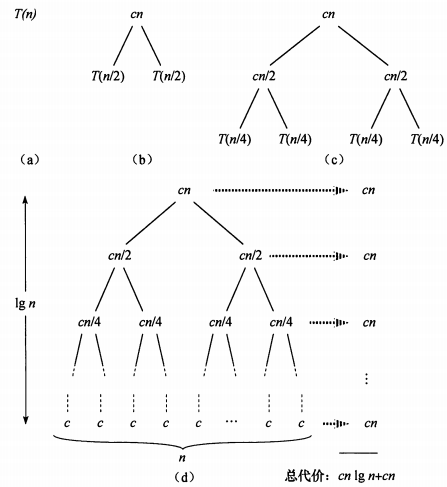在得到递归树后，将树中每层中的代价求和，得到每层代价，然后将所有层的代价求和，得到所有层次的递归调用的总代价。在上图(d)部分中，完全展开的递归树高度为lgnlg⁡n(树高为根结点到叶结点最长简单路径上边的数目)，所有递归树具有lgn+1lg⁡n+1层，所以总代价为cn∗(lgn+1)cn∗(lg⁡n+1)，所有时间复杂度为Θ(nlgn)Θ(nlg⁡n)。
总结：递归树模型求解递归方程，本质上就是迭代思想的应用，利用递归方程迭代展开过程构造对应的递归树，然后把每层的时间代价进行求和。不过递归树模型更直观，同时递归树也克服了二阶及更高阶递推方程不方便迭代展开的痛点。

4、主方法求解递推式
主方法为如下形式的递归式提供了一种“菜谱”式的求解方法，如下所示
T(n)=aT(n/b)+f(n)T(n)=aT(n/b)+f(n)

其中a≥1a≥1和b>1b>1是常数，f(n)f(n)是渐近正函数。这个递推式将规模为n的问题分解为a个子问题，每个子问题的规模为n/bn/b，a个子问题递归地求解，每个花费时间T(n/b)T(n/b)。函数f(n)f(n)包含了问题分解和子问题解合并的代价。同样，这个递归式也没有考虑上取整、下取整、边界条件等，结果不会影响递归式的渐近性质。

定理4.1(主定理) 令a≥1和b>1是常数，f(n)f(n)是一个函数，T(n)T(n)是定义在非负整数上的递归式：
T(n)=aT(n/b)+f(n)T(n)=aT(n/b)+f(n)
其中我们将n/bn/b解释为⌊n/b⌋⌊n/b⌋或⌈n/b⌉⌈n/b⌉。那么T(n)T(n)有如下渐近界：
1. 若对某个常数ε>0ε>0有f(n)=O(n(logba)−ε)f(n)=O(n(logb⁡a)−ε)，则T(n)=Θ(nlogba)T(n)=Θ(nlogb⁡a)
2. 若f(n)=Θ(nlogba)f(n)=Θ(nlogb⁡a)，则T(n)=Θ(nlogbalgn)T(n)=Θ(nlogb⁡alg⁡n)。
3. 若对某个常数ε>0ε>0有f(n)=Ω(n(logba)+ε)f(n)=Ω(n(logb⁡a)+ε)，且对某个常数c<1c<1和所有足够大的n有af(n/b)≤cf(n)af(n/b)≤cf(n)，则T(n)=Θ(f(n))T(n)=Θ(f(n))

在使用主定理之前，要比较f(n)和(nlogba)f(n)和(nlogb⁡a)的大小，这个大小不是算术意义上的大小比较，而是要在多项式意义上比较。以上三种情况在多项式意义上并未覆盖f(n)f(n)的所有可能性。情况1和情况2之间有一定间隙；情况2和情况3之间也有一定间隙。如果f(n)落在这两个间隙中，或者情况3中 正则条件不成立，就不能使用主方法来求递归式。    如在递归式：T(n)=2T(n/2)+nlgnT(n)=2T(n/2)+nlg⁡n中，因为 nlogba=n<f(n)=nlgnnlogb⁡a=n<f(n)=nlg⁡n，但是f(n)f(n)并不大于n一个多项式因子nεnε ,因为对于给定的ε>0ε>0当n足够大时，均有nε>lgnnε>lg⁡n。所以找不到这样 ε>0ε>0，该递归式落入了情况2和情况3之间的间隙，不能使用主定理。    最后给出主定理应用的几个练习题：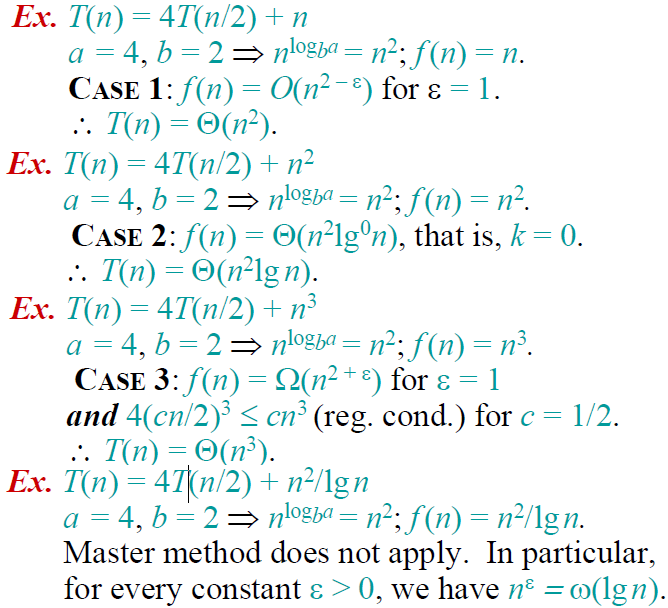具体举例分析：
【代入法】代入法首先要对这个问题的时间复杂度做出预测，然后将预测带入原来的递归方程，如果没有出现矛盾，则是可能的解，最后用数学归纳法证明。
【举   例】我们有如下的递归问题：T(n)=4T(n/2)+O(n)，我们首先预测时间复杂度为O(n2),不妨设T(n)=kn2（其中k为常数），将该结果带入方程中可得：左=kn2，右=4k(n/2)2+O(n)=kn2+O(n),由于n2的阶高于n的阶，因而左右两边是相等的，接下来用数学归纳法进行验证即可。

【迭代法】迭代法就是迭代的展开方程的右边，直到没有可以迭代的项为止，这时通过对右边的和进行估算来估计方程的解。比较适用于分治问题的求解，为方便讨论起见，给出其递归方程的一般形式：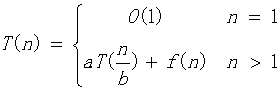【举   例】下面我们以一个简单的例子来说明：T(n)=2T(n/2)+n2,迭代过程如下：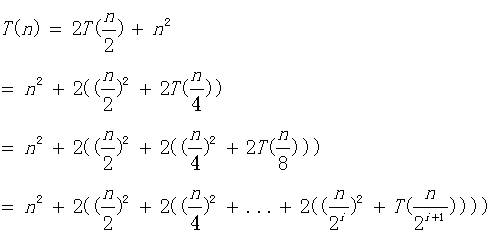容易知道，直到n/2^(i+1)=1时，递归过程结束，这时我们计算如下：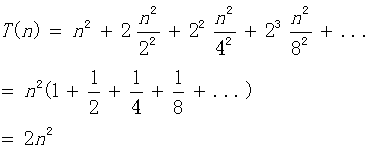到这里我们知道该算法的时间复杂度为O(n2)，上面的计算中，我们可以直接使用无穷等比数列的公式，不用考虑项数i的约束，实际上这两种方法计算的结果是完全等价的，有兴趣的同学可以自行验证。

【公式法】这个方法针对形如：T(n) = aT(n/b) + f(n)的递归方程。这种递归方程是分治法的时间复杂性所满足的递归关系，即一个规模为n的问题被分成规模均为n/b的a个子问题，递归地求解这a个子问题，然后通过对这a个子问题的解的综合，得到原问题的解。这种方法是对于分治问题最好的解法，我们先给出如下的公式：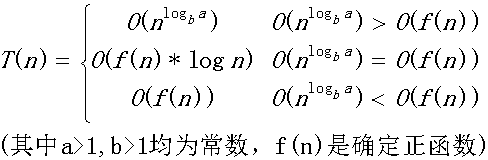公式记忆：我们实际上是比较n^logba和f(n)的阶，如果他们不等，那么T(n)取他们中的较大者，如果他们的阶相等，那么我们就将他们的任意一个乘以logn就可以了。按照这个公式，我们可以计算【迭代法】中提到的例子：O(f(n))=O(n2),容易计算另外一个的阶是O(n),他们不等，所以取较大的阶O(n2)。太简单了，不是吗？
需要注意：上面的公式并不包含所有的情况，比如第一种和第二种情况之间并不包含下面这种情况：f(n)是小于前者，但是并不是多项式的小于前者。同样后两种的情况也并不包含所有的情况。为了好理解与运用的情况下，笔者将公式表述成如上的情况，但是并不是很严谨，关于该公式的严密讨论，请看这里。但是公式的不包含的情况，我们很少很少碰到，上面的公式适用范围很广泛的。
特别地，对于我们经常碰到的，当f(n)=0时，我们有：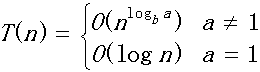【母函数法】母函数是用于对应于一个无穷序列的幂级数。这里我们解决的递归问题是形如：T(n)=c1T(n-1)+c2T(n-2)+c3T(n-3)+...+ckT(n-k)+f(n)。为说明问题简便起见，我们选择斐波那契数列的时间复杂度作为例子进行讨论。
【举  例】斐波那契数列递归公式：T(n)=T(n-1)+T(n-2)。这里我们假设F(n)为第n项的运算量。则容易得到：F(n)=F(n-1)+F(n-2),其中F(1)=F(2)=1.我们构造如下的母函数：G(x)=F(1)x+F(2)x2+F(3)x3+......,我们可以推导如下：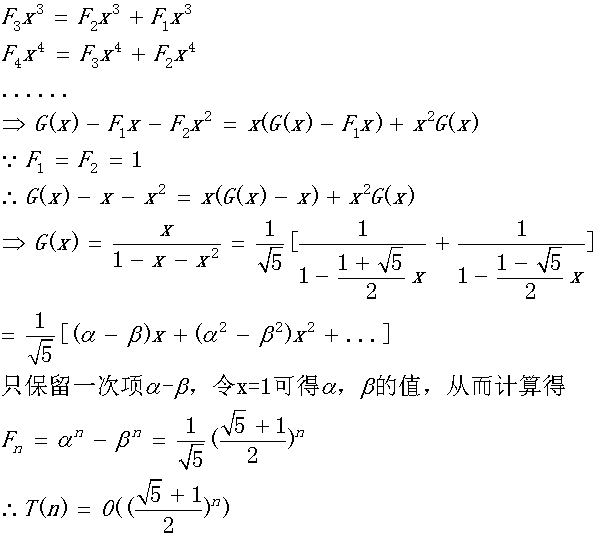上面的方法计算相对来说是比较简单的，关键在于对于母函数的理解，刚开始的时候可能不是很好理解，对于母函数可以参考这里和维基百科这里。

【差分方程法】可以将某些递归方程看成差分方程，通过解差分方程的方法来解递归方程，然后对解作出渐近阶估计。这里我们只考虑最长常见的递归形式，形如：T(n)=c1T(n-1)+c2T(n-2)+c3T(n-3)+...+ckT(n-k)+f(n)，其中c1,c2,...ck为常数且不等于0；我们对该方程的求解如下：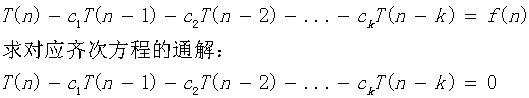对应上面的齐次方程的特征方程为：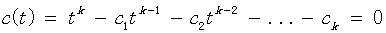如果解得t=r是该特征方程的m重根，则这m个解的形式为：{rn     n*rn      n2rn   ...    nm-1rn},其余的关于复数解的形式和普通的线性方程组的形式类似，不再赘述。接下来，我们要求解该方程的对应非齐次方程组的通解，这里我们针对该方程的特殊形式，不加证明地给出如下的通解形式：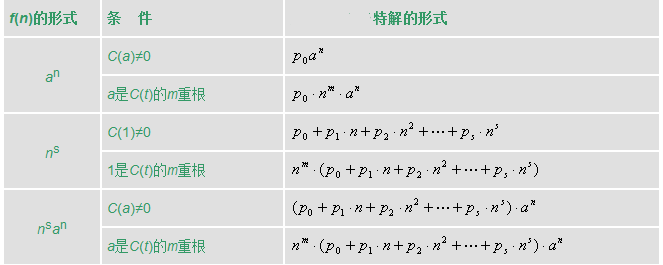则和线性代数中的解一样，原方程的解等于齐次方程组的通解+特解，即：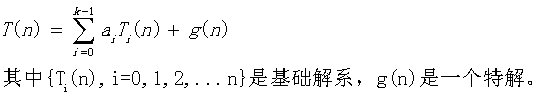最后由初始条件确定a(i)的值即可。
为了帮助理解，我们举两个例子看看，就明白是怎么回事了。
【举 例1】递归方程如下：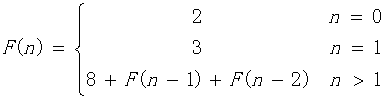(1)写出对应齐次方程的特征方程：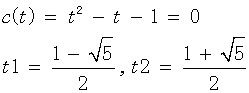得到基础解系为：{t1n,  t2n}
(2)计算特解，对于本题，直接观察得特解为：-8
(3)得到原方程解的形式为：T(n)=a0t1n+a1t2n-8
(4)代入n=0，n=1的情况，得到a0,a1，最后可得: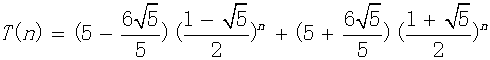可以看到该方程形式和上面讨论过的斐波那契数列只差一个常数8，因而两者的时间复杂度是相同的。有兴趣的同学可以按照这个方法再次计算斐波那契数列的时间复杂度验证一下。
【举  例2】递归方程如下：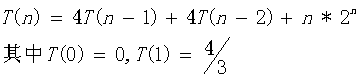（1）计算对应齐次方程的基础解析：特征方程为：C(t)=t^2-4t-4=0,得到一个2重根t=2.因而其基础解为：{2n      n*2n}
（2）由于f(n)=n*2n，对应上面表格的最后一种情况，得到特解形式为：T(n)=n2(p0+p1n)2n代入原递归方程可得：p0=1/2,p1=1/6
（3）原方程解的形式为：T(n)=a0*2n+a1*n*2n+n2(1/2+n/6)2n,代入T(0),T(1)得：a0=a1=0
（4）综上：T(n)=n2(1/2+n/6)2n
因而时间复杂度为：O(n32n).

参考：https://blog.csdn.net/so_geili/article/details/53444816
https://blog.csdn.net/m0_37734999/article/details/78751882
https://www.cnblogs.com/hlongch/p/5749038.html
展开全文• 其实，只要讲到数据结构与算法，就一定离不开时间、空间复杂度分析。而且我个人认为，复杂度分析是整个算法学习的精髓，只要掌握了它，数据结构和算法的内容基本上就掌握了一半。 1.时间复杂度分析 对于刚才...
    其实，只要讲到数据结构与算法，就一定离不开时间、空间复杂度分析。而且我个人认为，复杂度分析是整个算法学习的精髓，只要掌握了它，数据结构和算法的内容基本上就掌握了一半。

1.时间复杂度分析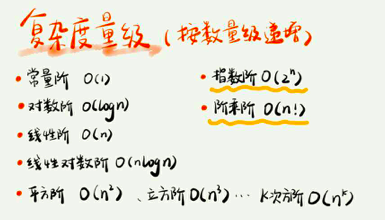对于刚才罗列的复杂度量级，我们可以粗略地分为两类，多项式量级和非多项式量级。其中，非多项式量级只有两个：O(2n) 和 O(n!)。当数据规模 n 越来越大时，非多项式量级算法的执行时间会急剧增加，求解问题的执行时间会无限增长。所以，非多项式时间复杂度的算法其实是非常低效的算法。

2.空间复杂度分析
时间复杂度的全称是渐进时间复杂度，表示算法的执行时间与数据规模之间的增长关系。类比一下，空间复杂度全称就是渐进空间复杂度（asymptotic space complexity），表示算法的存储空间与数据规模之间的增长关系。

3. 最好，最坏，平均，均摊时间复杂度
3.1 复杂度分析的4个概念
• 最坏情况时间复杂度:代码在最理想情况下执行的时间复杂度。
• 最好情况时间复杂度:代码在最坏情况下执行的时间复杂度。
• 平均时间复杂度:用代码在所有情况下执行的次数的加权平均值表示。
• 均摊时间复杂度:在代码执行的所有复杂度情况中绝大部分是低级别的复杂度,个别情况是高级别复杂度且发生具有时序关系时,可以将个别高级别复杂度均摊到低级 别复杂度上。基本上均摊结果就等于低级别复杂度。
3.2 为什么要引入这4个概念?
• 同一段代码在不同情况下时间复杂度会出现量级差异,为了更全面,更准确的描述代码的时间复杂度,所以引入这4个概念。
• 代码复杂度在不同情况下出现量级差别时才需要区別这四种复杂度。大多数情况下,是不需要区别分析它们的。
3.3 如何分析平均、均摊时间复杂度?
• 平均时间复杂度：代码在不同情况下复杂度出现量级差别,则用代码所有可能情况下执行次数的加权平均值表示。
• 均摊时间复杂度：两个条件满足时使用:1)代码在绝大多数情况下是低级 别复杂度,只有极少数情况是高级别复杂度;2)低级别和高级别复杂度出现具有时序规律。均摊结果一般都等于低级别复杂度.

4.内容小结
复杂度也叫渐进复杂度，包括时间复杂度和空间复杂度，用来分析算法执行效率与数据规模之间的增长关系，可以粗略地表示，越高阶复杂度的算法，执行效率越低。常见的复杂度并不多，从低阶到高阶有：O(1)、O(logn)、O(n)、O(nlogn)、O(n2 )。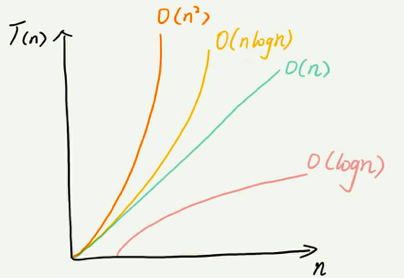展开全文数据结构 数组
• int f(unsigned int n) { if(n <= 1) { return 1; } return f(n-1) + f(n-2); }shell
• 改进的堆排序算法及其复杂度分析改进的堆排序算法及其复杂度分析改进的堆排序算法及其复杂度分析改进的堆排序算法及其复杂度分析
• 算法的复杂度分析算法的复杂度分析插入排序为例 算法的复杂度分析 插入排序为例


算法的复杂度分析
算法的复杂度分析插入排序为例

算法的复杂度分析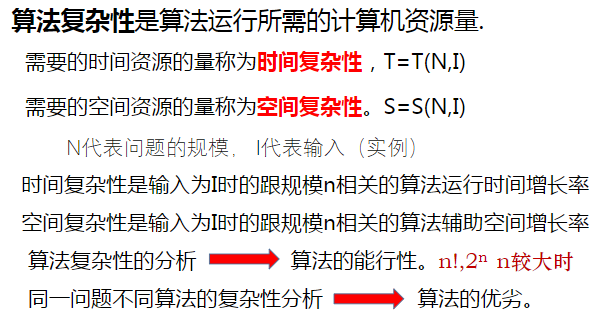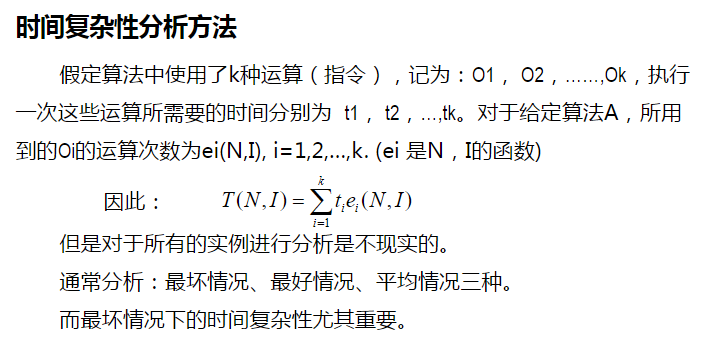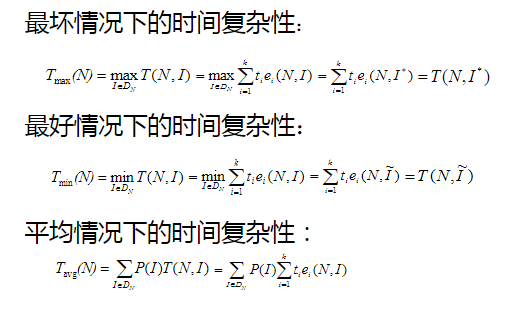插入排序为例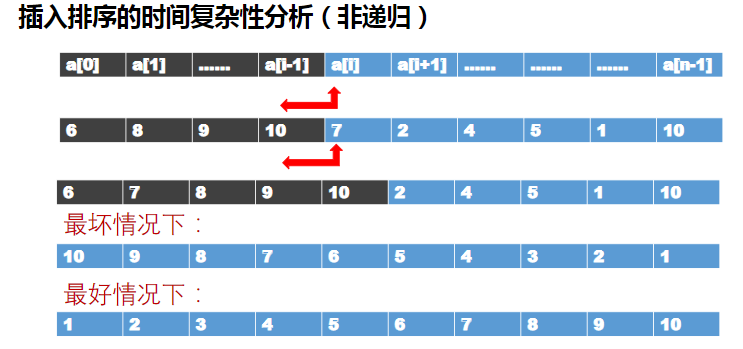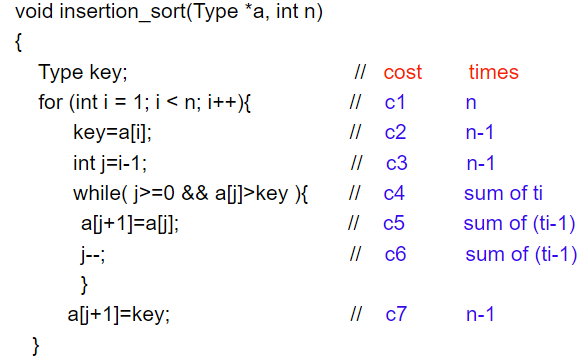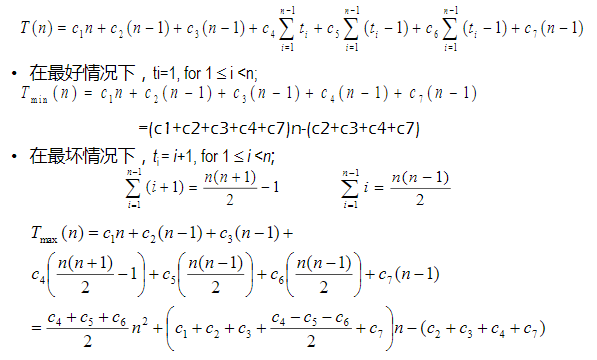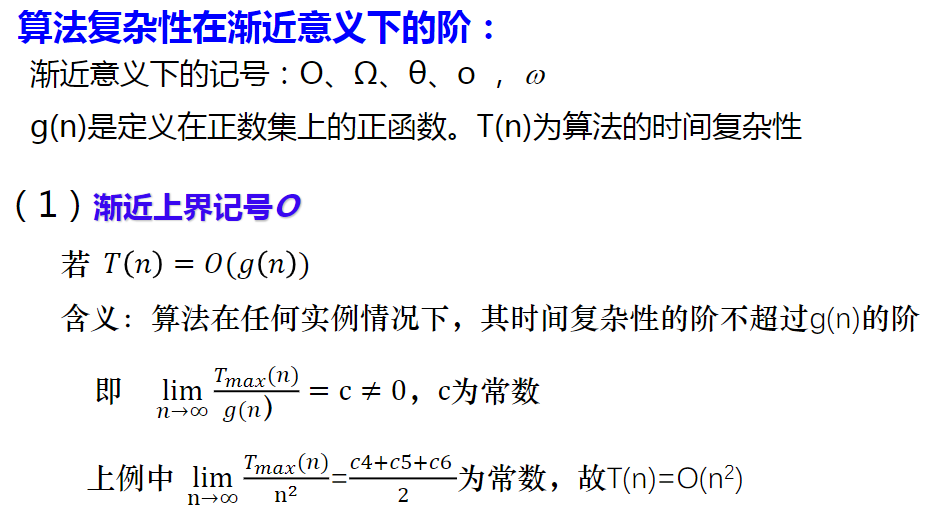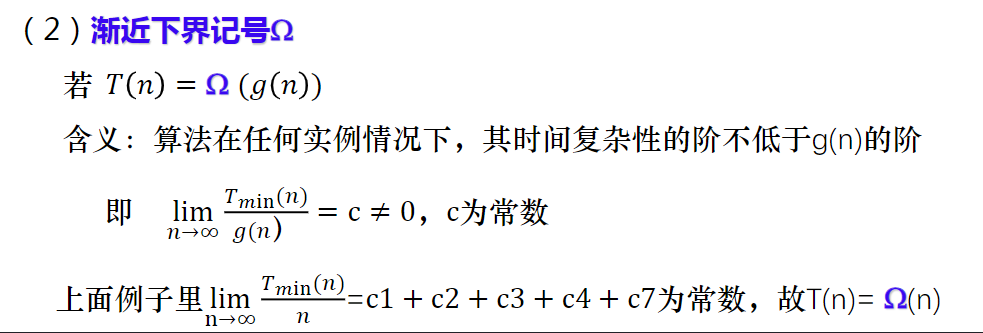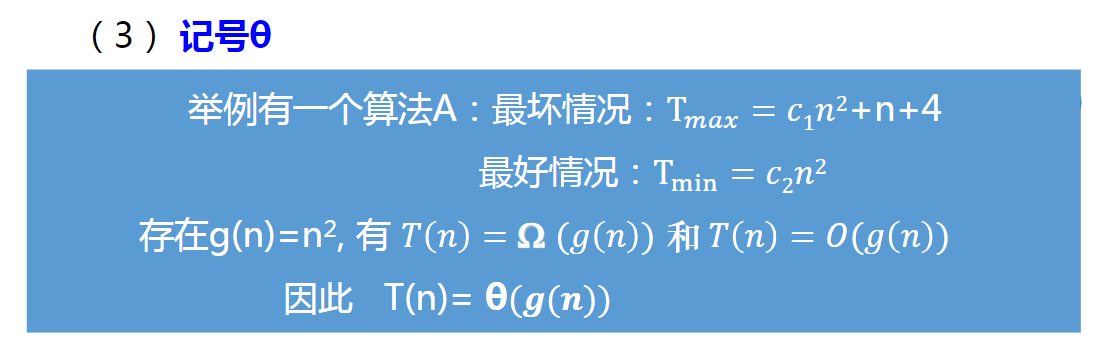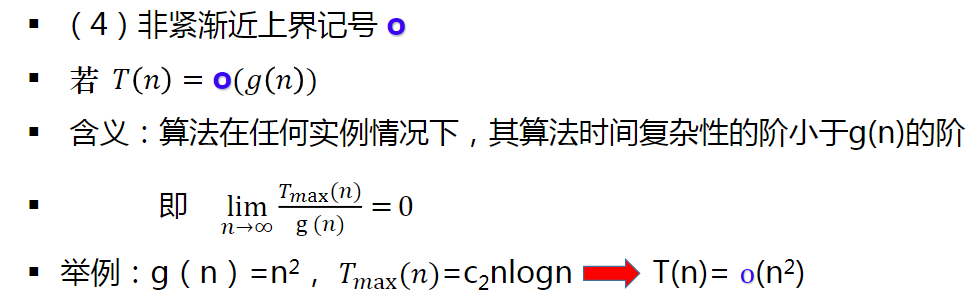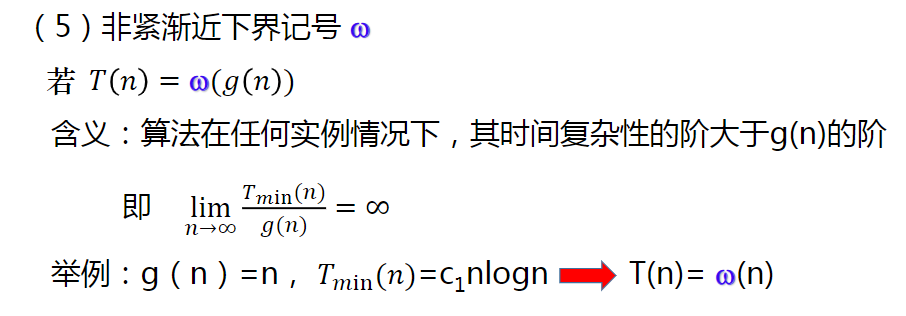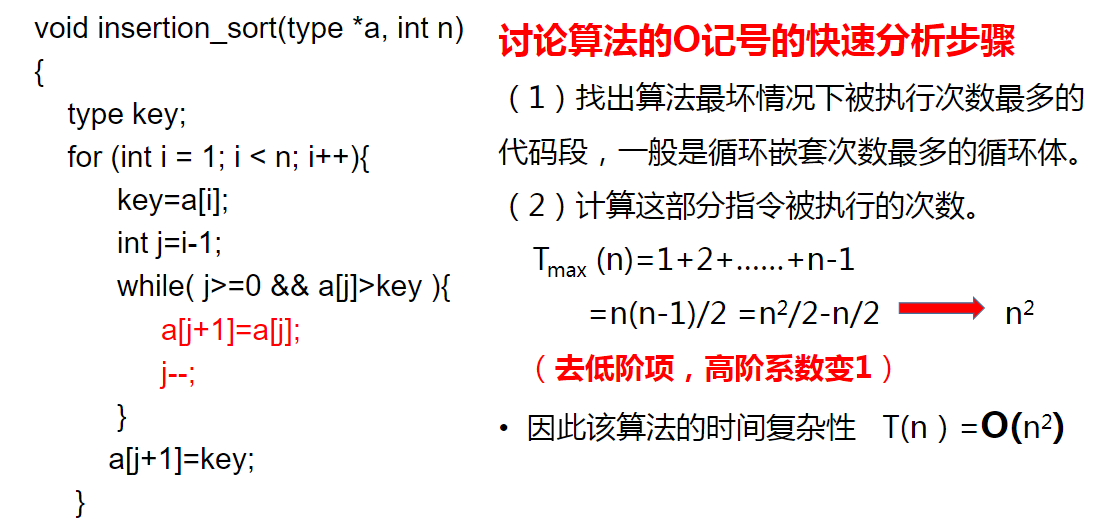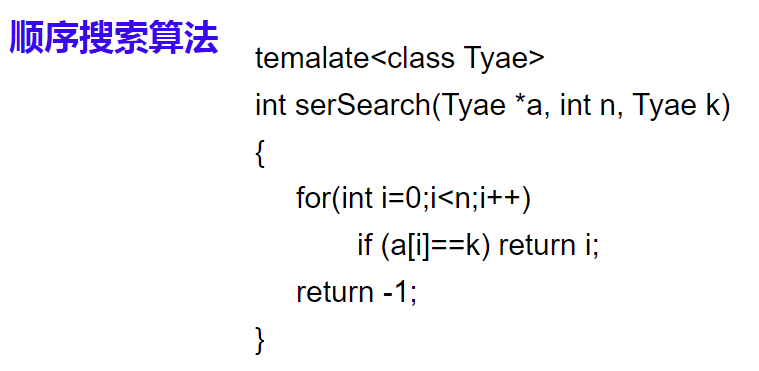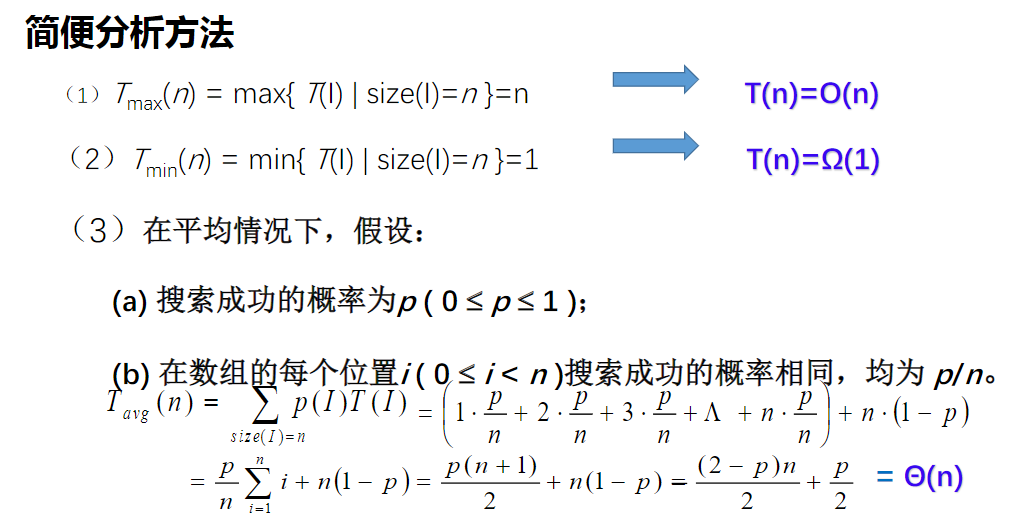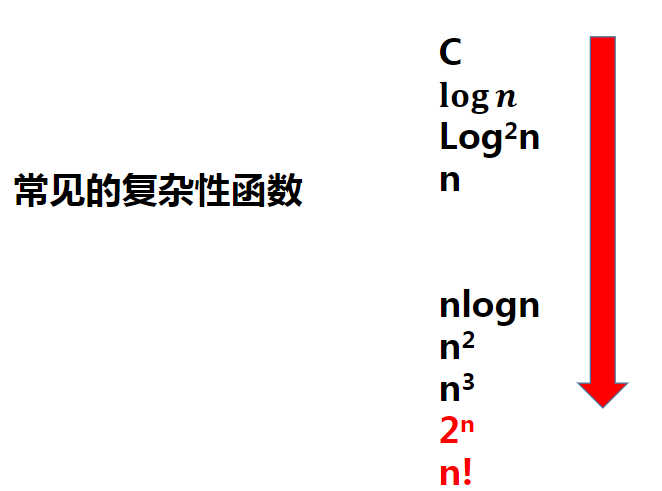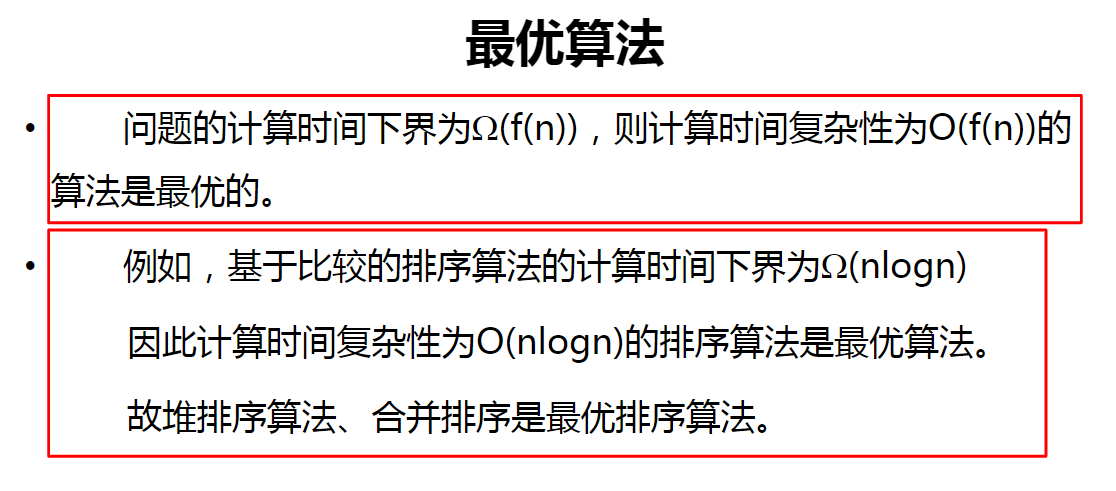展开全文...